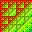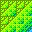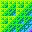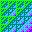Sparse Arrays in J Copyright © 1998, 1999, Iverson Software Inc.Introduction

We describe a sparse array extension to J using a representation that “does not store zeros”. One new verb \$. is defined to create and manipulate sparse arrays, and existing primitives are extended to operate on such arrays. These ideas are illustrated in following examples:

 ``` ] d=: (?. 3 4\$2) * ?. 3 4\$100 0 75 0 53 0 0 67 67 93 0 51 83 ```

 ``` ] s=: \$. d 0 1 | 75 0 3 | 53 1 2 | 67 1 3 | 67 2 0 | 93 2 2 | 51 2 3 | 83 ``` convert d to sparse and assign to s the display of s gives the indices of the “non-zero” cells and the corresponding values

 ``` d -: s 1 ``` d and s match

 ``` o. s 0 1 | 235.619 0 3 | 166.504 1 2 | 210.487 1 3 | 210.487 2 0 | 292.168 2 2 | 160.221 2 3 | 260.752 ``` π times s

 ``` o. d 0 235.619 0 166.504 0 0 210.487 210.487 292.168 0 160.221 260.752 ``` π times d

 ``` (o. s) -: o. d 1 ``` function results independent of representation

 ``` 0.5 + o. s 0 1 | 236.119 0 3 | 167.004 1 2 | 210.987 1 3 | 210.987 2 0 | 292.668 2 2 | 160.721 2 3 | 261.252 <. 0.5 + o. s 0 1 | 236 0 3 | 167 1 2 | 210 1 3 | 210 2 0 | 292 2 2 | 160 2 3 | 261 (<. 0.5 + o. s) -: <. 0.5 + o. d 1 ```

 ``` d + s 0 1 | 150 0 3 | 106 1 2 | 134 1 3 | 134 2 0 | 186 2 2 | 102 2 3 | 166``` function arguments can be dense or sparse

 ``` (d + s) -: 2*s 1 (d + s) -: 2*d 1 +/ s 0 | 93 1 | 75 2 | 118 3 | 203 +/"1 s 0 | 128 1 | 134 2 | 227 ``` familiar algebraic properties are preserved

 ``` |. s 0 0 | 93 0 2 | 51 0 3 | 83 1 2 | 67 1 3 | 67 2 1 | 75 2 3 | 53 |."1 s 0 0 | 53 0 2 | 75 1 0 | 67 1 1 | 67 2 0 | 83 2 1 | 51 2 3 | 93 ``` reverse

 ``` |: s 0 2 | 93 1 0 | 75 2 1 | 67 2 2 | 51 3 0 | 53 3 1 | 67 3 2 | 83 \$ |: s 4 3 ``` transpose
 ``` \$.^:_1 |: s 0 0 93 75 0 0 0 67 51 53 67 83 (|:s) -: |:d 1 ``` \$.^:_1 converts a sparse array to dense
 ``` , s 1 | 75 3 | 53 6 | 67 7 | 67 8 | 93 10 | 51 11 | 83 \$ , s 12 ``` ravel; a sparse vector

To the top.Representation

A sparse array y may be boolean, integer, floating point, complex, literal, or boxed, and has the (internal) parts sh;a;e;i;x;flag where:

 sh Shape, \$y . Elements of the shape must be less than 2^31 , but the product over the shape may be larger than 2^31 . sh Axe(s), a vector of the sorted sparse (indexed) axes. e Sparse element (“zero”). e is also used as the fill in any overtake of the array. i Indices, an integer matrix of indices for the sparse axes. x Values, a (dense) array of usually non-zero cells for the non-sparse axes corresponding to the index matrix i . flag Various bit flags.

For the sparse matrix s used in the introduction,

```   ] d=: (?. 3 4\$2) * ?. 3 4\$100
0 75  0 53
0  0 67 67
93  0 51 83

] s=: \$. d
0 1 | 75
0 3 | 53
1 2 | 67
1 3 | 67
2 0 | 93
2 2 | 51
2 3 | 83
```

The shape is 3 4 ; the sparse axes are 0 1 ; the sparse element is 0 ; the indices are the first two columns of numbers in the display of s ; and the values are the last column.

Scalars continue to be represented as before (densely). All primitives accept sparse or dense arrays as arguments (e.g. sparse+dense or sparse\$sparse). The display of a sparse array is a display of the index matrix (the i part), a blank column, a column of vertical lines, another blank column, and the corresponding value cells (the x part).

Letting the sparse element be variable rather than fixed at zero makes many more functions closed on sparse arrays (e.g. ^y or 10+y or -.y), and familiar results can be produced by familiar phrases (e.g. <.0.5+y for rounding to the nearest integer).

To the top.Assertions

The following assertions hold for a sparse array, and displaying a sparse array invokes these consistency checks on it.

 imax =: _1+2^31 the largest internal integer rank =: #@\$ rank type =: 3!:0 internal type 1 = rank sh vector sh -: <. sh integral imax >: #sh at most imax elements (0<:sh) *. (sh<:imax) bounded by 0 and imax 1 = rank a vector a e. i.#sh bounded by 0 and rank-1 a -: ~. a elements are unique a -: /:~ a sorted 0 = rank e atomic (type e) = type x has the same internal type as x 2 = rank i matrix 4 = type i integral (#i) = #x as many rows as the number of items in x ({:\$i) = #a as many columns as there are sparse axes (#i) <: */a{sh # rows bounded by product over sparse axes lengths imax >: */\$i # elements is bounded by imax (0<:i) *. (i <"1 a{sh) bounded by 0 and the lengths of the sparse axes i -: ~.i rows are unique i -: /:~ i rows are sorted (rank x) = 1+(#sh)-#a rank equals 1 plus the number of dense axes imax >: */\$x # elements is bounded by imax (}.\$x)-:((i.#sh)-.a){sh item shape is the dimensions of the dense axes (type x) e. 1 2 4 8 16 32 internal type is boolean, character, integer, real, complex, or boxed

To the top.The Verb \$.

The ranks of \$. are infinite. The inverse of n&\$. is (-n)&\$. .

\$.y converts a dense array to sparse, and conversely \$.^:_1 y converts a sparse array to dense. The identities f -: f&.\$. and f -: f&.(\$.^:_1) hold for any function f, with the possible exception of those (like overtake {.) which use the sparse element as the fill.

0\$.y applies \$. or \$.^:_1 as appropriate; that is, converts a dense array to sparse and a sparse array to dense.

1\$.sh;a;e produces a sparse array. sh specifies the shape. a specifies the sparse axes; negative indexing may be used. e specifies the “zero” element, and its type determines the type of the array. The argument may also be sh;a (e is assumed to be a floating point 0) or just sh (a is assumed to be i.#sh — all axes are sparse — and e a floating point 0).

2\$.y gives the sparse axes (the a part);
(2;a)\$.y (re-)specifies the sparse axes;
(2 1;a)\$.y
gives the number of bytes required for (2;a)\$.y ;
(2 2;a)\$.y
gives the number of items in the i part or the x part for the specified sparse axes a (that is, #4\$.(2;a)\$.y).

3\$.y gives the sparse element (the e part); (3;e)\$.y respecifies the sparse element.

4\$.y gives the index matrix (the i part).

5\$.y gives the value array (the x part).

6\$.y gives the flag (the flag part).

7\$.y gives the number of non-sparse entries in array y ; that is, #4\$.y or #5\$.y .

8\$.y removes any completely “zero” value cells and the corresponding rows in the index matrix.Further Examples

 ``` ] d=: (0=?. 2 3 4\$3) * ?. 2 3 4\$100 13 0 0 0 21 4 0 0 0 0 0 0 3 5 0 0 0 0 6 0 0 0 0 0 ```

 ``` ] s=: \$. d 0 0 0 | 13 0 1 0 | 21 0 1 1 | 4 1 0 0 | 3 1 0 1 | 5 1 1 2 | 6 ``` convert d to sparse and assign to s

 ``` d -: s 1 ``` match is independent of representation

 ``` 2 \$. s 0 1 2 ``` sparse axes

 ``` 3 \$. s 0 ``` sparse element

 ``` 4 \$. s 0 0 0 0 1 0 0 1 1 1 0 0 1 0 1 1 1 2 ``` index matrix; columns correspond to the sparse axes

 ``` 5 \$. s 13 21 4 3 5 6 ``` corresponding values

 ``` ] u=: (2;2)\$.s 0 | 13 21 0 | 3 0 0 | 1 | 0 4 0 | 5 0 0 | 2 | 0 0 0 | 0 6 0 ``` make 2 be the sparse axis

 ``` 4 \$. u 0 1 2 ``` index matrix

 ``` 5 \$. u 13 21 0 3 0 0 0 4 0 5 0 0 0 0 0 0 6 0 ``` corresponding values

 ``` ] t=: (2;0 1)\$.s 0 0 | 13 0 0 0 0 1 | 21 4 0 0 1 0 | 3 5 0 0 1 1 | 0 0 6 0 ``` make 0 1 be the sparse axes

 ``` 7 {. t 0 0 | 13 0 0 0 0 1 | 21 4 0 0 1 0 | 3 5 0 0 1 1 | 0 0 6 0 \$ 7 {. t 7 3 4 ``` take

 ``` 7 {."1 t 0 0 | 13 0 0 0 0 0 0 0 1 | 21 4 0 0 0 0 0 1 0 | 3 5 0 0 0 0 0 1 1 | 0 0 6 0 0 0 0 0 = t 0 0 | 0 1 1 1 0 1 | 0 0 1 1 1 0 | 0 0 1 1 1 1 | 1 1 0 1 ``` take with rank

 ``` 3 \$. 0 = t 1 +/ , 0 = t 18 ``` the sparse element of 0=t is 1

 ``` +/ , 0 = d 18 ``` answers are independent of representation

 ``` 0{t 0 | 13 0 0 0 1 | 21 4 0 0 ``` from

 ``` _2 (<1 2 3)}t 0 0 | 13 0 0 0 0 1 | 21 4 0 0 1 0 | 3 5 0 0 1 1 | 0 0 6 0 1 2 | 0 0 0 _2 ``` amend

 ``` ] p=: (i.!n) A. i.n=: 3 0 1 2 0 2 1 1 0 2 1 2 0 2 0 1 2 1 0 ``` all permutations of order 3

 ``` C.!.2 p 0 1 1 0 0 1 ``` the parity of each permutation

 ``` \$.^:_1 (_1^C.!.2 p) (<"1 p)} 1 \$. n\$n 0 0 0 0 0 1 0 _1 0 0 0 _1 0 0 0 1 0 0 0 1 0 _1 0 0 0 0 0 ```

The last expression computes the complete skewed tensor of order 3.

 ``` s=: 1 \$. 20 50 1000 75 366 ```

 ``` \$s 20 50 1000 75 366 ``` 20 countries, 50 regions, 1000 salespersons, 75 products, 366 days in a year

 ``` */ \$s 2.745e10 ``` the product over the shape can be greater than 2^31

 ``` r=: ?. 1e5 \$ 1e6 i=: ?. 1e5 5 \$ \$ s s=: r (<"1 i)} s ``` revenues corresponding locations assign revenues to corresponding locations

 ``` 7 {. ": s 0 0 5 30 267 | 128133 0 0 26 20 162 | 319804 0 0 31 37 211 | 349445 0 0 37 10 351 | 765935 0 0 56 6 67 | 457449 0 0 66 54 120 | 38186 0 0 71 74 246 | 515473 ``` the first 7 rows in the display of s ; the first row says that for country 0, region 0, salesperson 5, product 30, day 267, the revenue was 128133

 ``` +/ , s |limit error | +/ ,s ``` total revenue the expression failed on ,s because it would have required a vector of length 2.745e10

 ``` +/@, s 5.00289e10 ``` total revenue f/@, is supported by special code

 ``` +/+/+/+/+/ s 5.00289e10 +/^:5 s 5.00289e10 +/^:_ s 5.00289e10 +/ r 5.00289e10 ``` total revenue

 ``` +/"1^:4 s 0 | 2.48411e9 1 | 2.55592e9 2 | 2.55103e9 3 | 2.52089e9 4 | 2.49225e9 5 | 2.45682e9 6 | 2.52786e9 7 | 2.45425e9 8 | 2.48729e9 9 | 2.50094e9 10 | 2.51109e9 11 | 2.59601e9 12 | 2.49003e9 13 | 2.58199e9 14 | 2.44772e9 15 | 2.47863e9 16 | 2.46455e9 17 | 2.5568e9 18 | 2.43492e9 19 | 2.43582e9 ``` total revenue by country

 ``` t=: +/^:2 +/"1^:2 s \$t 1000 7{.t 0 | 4.58962e7 1 | 4.81548e7 2 | 3.97248e7 3 | 4.89981e7 4 | 4.85948e7 5 | 4.69227e7 6 | 4.22094e7 ``` total revenue by salesperson

To the top.Sparse Linear Algebra

Currently, only sparse matrix multiplication and the solutions of tri-diagonal linear system are implemented. For example:

 ``` f=: }. @ }: @ (,/) @ (,."_1 +/&_1 0 1) @ i. ```

 ``` f 5 0 1 1 0 1 1 1 2 2 1 2 2 2 3 3 2 3 3 3 4 4 3 4 4 ``` indices for a 5 by 5 tri-diagonal matrix

 ``` s=: (?. 13\$100) (<"1 f 5)} 1 \$. 5 5;0 1 \$s 5 5 ```

The phrase 1\$.5 5;0 1 makes a sparse array with shape 5 5 and sparse axes 0 1 (sparse in both dimensions); <"1 f 5 makes boxed indices; and x (<"1 f 5)}y amends by x the locations in y indicated by the indices (scattered amendment).

 ``` s 0 0 | 13 0 1 | 75 1 0 | 45 1 1 | 53 1 2 | 21 2 1 | 4 2 2 | 67 2 3 | 67 3 2 | 93 3 3 | 38 3 4 | 51 4 3 | 83 4 4 | 3 ```

 ``` ] d=: \$.^:_1 s 13 75 0 0 0 45 53 21 0 0 0 4 67 67 0 0 0 93 38 51 0 0 0 83 3 ``` the dense representation of s

 ``` ] y=: ?. 5\$80 10 60 36 42 17 y %. s 1.27885 _0.0883347 0.339681 0.202906 0.0529263 ```

 ``` y %. d ``` answers are independent of representation 1.27885 _0.0883347 0.339681 0.202906 0.0529263

 ``` s=: (?. (_2+3*1e5)\$1000) (<"1 f 1e5)} 1 \$. 1e5 1e5;0 1 ```

 ``` \$s 100000 100000 y=: ?. 1e5\$1000 ``` s is a 1e5 by 1e5 matrix

 ``` ts=: 6!:2 , 7!:2@] ``` time and space for execution

 ``` ts 'y %. s' 0.28 5.2439e6 ``` 0.28 seconds; 5.2 megabytes (Pentium 266 Mhz)

To the top.Implementation Status

As of 1999-09-03 12:00 , the following facilities support sparse arrays:

 = d =. =: < <. <: > >. >: _ _. _: + +. +: * *. *: - -. m -: % %. d %: ^ ^. \$ m \$. \$: ~ ~: d | |. |: .. .: : :. :: , ,. ,: # ! !. !: / m \ m \. m [ [. [: ] ]. { d {. {: } d }. }: " ". ": m ` `: @ @. @: & &. &: j. m o. r. m _9: to 9: 3!:0 3!:1 3!:2 3!:3 4!:55

Notes:

 • Sparse literal and boxed arrays not yet implemented. • The dyad %. only implements the case of triadiagonal matrices. • Boxed left arguments for |: (diagonal slices) not yet implemented. • The monads f/ and f/"r are only implemented for + * >. <. +. *. = ~: , (and only boolean arguments for = and ~:); on an axis of length 2, the monads f/ and f/"r are implemented for any function. • { and } only accept the following index arguments: integer arrays, <"1 on integer arrays, and scalar boxed indices (respectively, item indexing, scattered indexing, and index lists a0;a1;a2;…).

To the top.

 created: 1998-11-13 07:10 updated: 2019-10-22 23:00